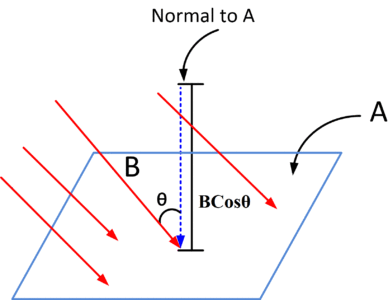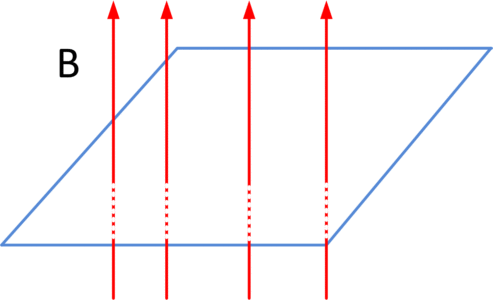Home / Magnetism / Magnetic Flux Definition and Unit

# Magnetic Flux Definition and Unit

Want create site? Find Free WordPress Themes and plugins.

Magnetic Flux is defined as;

“a total number of lines of magnetic force passing through any surface placed perpendicular to the magnetic field. It is denoted by φ (Greek Letters Phi).

Magnetic Flux Formula

The magnetic flux is also defined as the dot product of magnetic field B and vector Area A, as shown in the following figure.$\text{Magnetic }\!\!~\!\!\text{ Flux}=\text{ }\!\!\varphi\!\!\text{ }=B.A~~~\text{ }\cdots \text{ }~~~~~\left( 1 \right)$

Where θ is the angle between B and vector area A or the outward normal drawn to the surface area as shown in above figure. A is a vector area whose magnitude is the area of the element and its direction is along the normal to the surface of the element.

Maximum Flux

If the magnetic field is directed along the normal to the area, so θ is 0 as shown in the following figure.Now by equation (1), we get

$\varphi =BAcos~\left( {{0}^{}} \right)$

Hence

$\varphi =BA~~~~\cdots \text{ }~~~~\left( 2 \right)$

Equation (2) shows that the magnetic flux is maximum when the angle between a magnetic field and normal to an area is zero.

Minimum Flux

If the magnetic field is parallel to the plane of area and angle between the field and normal to an area is 90°   as shown in the figure.Then

$\varphi =BAcos~\left( {{90}^{o}} \right)$

$\varphi =0$

It means that flux through an area in this position is zero or minimum.

## Magnetic Flux Unit

SI unit of magnetic flux is Weber, as explained below,

$\varphi =BAcos\theta$

$\varphi =BA~~~~\text{ }~~\therefore ~~surface~area~perpendicular~to~B,~so~Cos\theta =1~~$

$\varphi =\frac{Newton}{Ampere-meter}*~mete{{r}^{2}}$

$\varphi =\frac{N}{A-m}*~{{m}^{2}}$

$\varphi =\frac{Nm}{A}$

$\varphi =Weber$

Hence magnetic flux unit is  NmA-1 or weber. Whereas

$1\text{ }Weber={{10}^{8}}lines\text{ }of\text{ }force$

Example

A certain magnet has a flux φ = 1.5 Weber. How many lines of force does flux represent?

Solution:

By definition,

$1\text{ }Weber={{10}^{8}}lines\text{ }of\text{ }force$

So,

$1.5\text{ }Weber\text{ }=\text{ }1.5\text{ }*\text{ }{{10}^{8}}lines$

You May Also Read:

Magnetomotive Force and Unit

Magnetic Flux Density Definition and Unit

Magnetic Flux Intensity Definition and Unit

Did you find apk for android? You can find new Free Android Games and apps.

### About Ahmad FaizanMr. Ahmed Faizan Sheikh, M.Sc. (USA), Research Fellow (USA), a member of IEEE & CIGRE, is a Fulbright Alumnus and earned his Master’s Degree in Electrical and Power Engineering from Kansas State University, USA.